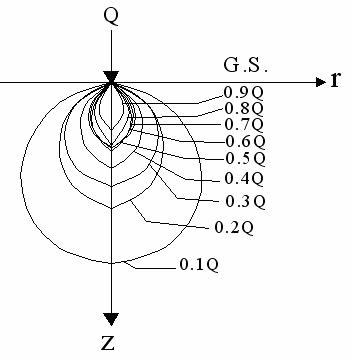Foundation, Concrete and Earthquake Engineering

### Different Types of Earth Pressure Cells, Their Applications and Construction of Isobar Diagram Using Them-3

1.4 ISOBAR DIAGRAM
An isobar is a curve joining the points of equal stress intensity. In other words, an isobar is a contour of equal stress. An isobar is a spatial curved surface of the shape of an electrical bulb or an onion. The curved surface is symmetrical about the vertical axis passing through the load point.

The isobar of a particular intensity can be obtained using Boussinesq’s equation. The calculations are shown in a tabular form. Table 1.1. gives calculations for an isobar of intensity 0.1Q per unit area.Fig.1.3: Pressure Bulb or Isobar Diagram for Point Load Q.
From Boussinesq’s equation,
σz = IB . Q /z 2

Taking σz = 0.1Q

0.1Q = IB .Q/z2
or, IB =0.1 z2 …………………….(1.2)

For different depths z, the value of IB is computed from Eq. 1.2. as shown in second row of Table 1.1. The values of r/z for computed values of IB are obtained from Eq.1.4. Once the values of r/z have been determined, the radial distance r can be obtained as shown in table 1.1. It may be observed that r is zero at the load point, and it attains a maximum value at r/z = 0.75 and again decreases. As the isobar is symmetrical about the load axis, the other half can be drawn from symmetry. The shape of an isobar approaches a lemniscates curve. Fig. 1.3. shows the isobar diagram or pressure bulb for point load.

In the similar manner, the isobars of other intensity 0.2Q, 0.3Q, 0.4Q, etc. can be drawn. Obviously, the isobars of higher intensity shall lie within the isobar of 0.1Q.

Table 1.1- Calculations for Isobar of 0.1 Q
 Depth z 0.25 0.5 0.75 1 1.25 1.5 1.75 2 2.185 IB 0.00625 0.25 0.05625 0.1 0.1562 0.225 0.3062 0.4 0.4775 r/z 2.16 1.5 1.16 0.93 0.75 0.59 0.44 0.27 0 r 0.54 0.75 0.87 0.93 0.938 0.885 0.77 0.54 0

Isobars are useful for determining the effect of the load on the vertical stresses at various points. The zone within which the stresses have a significant effect on the settlement of structures is known as the Pressure Bulb. The area outside the pressure bulb is assumed to have negligible stresses.